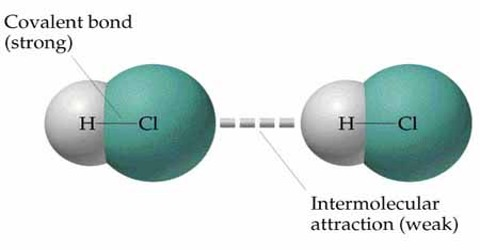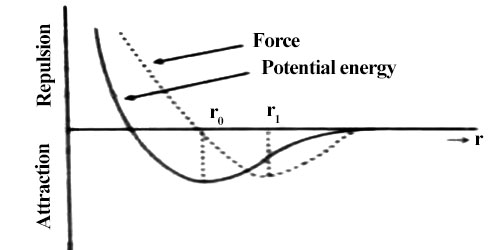# Explanation of Elasticity in the Light of Intermolecular Force

Elasticity in the light of intermolecular force

When force is applied on a crystalline body, atoms/molecules of the body are displaced from their equilibrium positions. But these molecules always tend to return to their original stable positions. So, a restoring force is generated against this displacement. By applying force if a body is expanded then restoring force becomes attractive; on the other hand if external force tries to contract a body, the restoring force becomes repulsive. For equilibrium state to attain the applied force must be equal to the restoring force. This restoring force is called elastic force. For the same amount of displacement of different bodies elastic force is not same. For that reason elasticity of different materials is different.

If the applied force for expansion or contraction of a body is not large, then displacement becomes linear. In figure, position slightly above or slightly below r0 may be considered as linear. In this situation elastic force is proportional to displacement. If applied force is withdrawn then the body returns to its original shape due to elastic force.From figure, it is seen that if the intermolecular distance, is more than r1, the magnitude of force starts decreasing i.e., attractive force becomes decreasing. In this condition, if the applied force is withdrawn the body does not go back to its original shape. Then a permanent deformation occurs in the body that means the body loses elasticity. So, it is seen that there is a maximum limit of applied force. Upto that limit the body remains elastic for applied force, that means it the applied force is withdrawn the body regains its original shape; but if the limit is exceeded the body loses its elastic behavior. This limit is called the elastic limit.